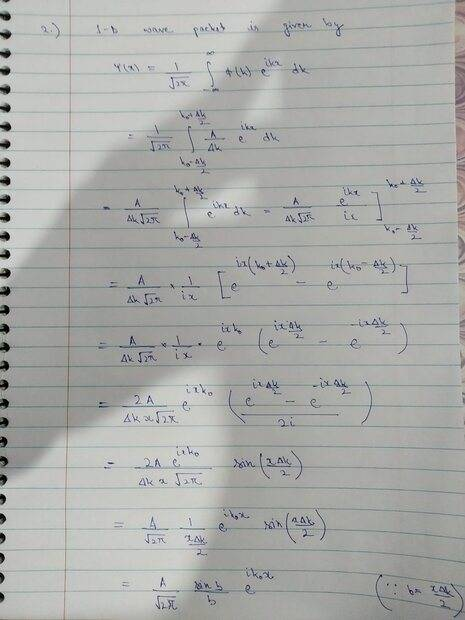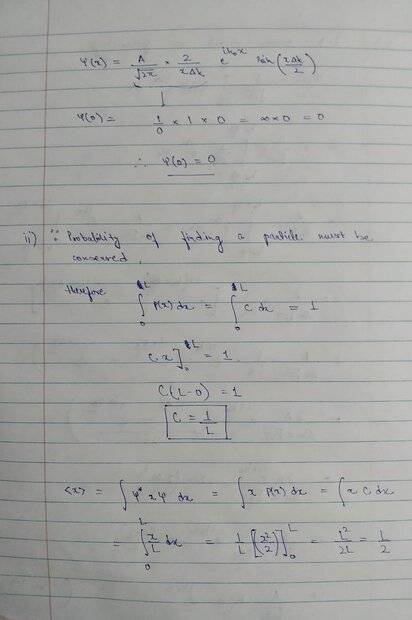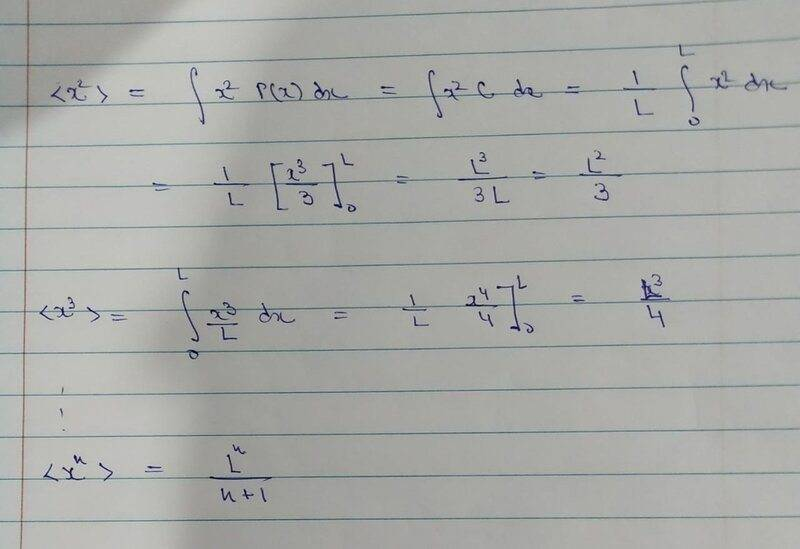# [Intro QM] Verification requested on possible solution for a question

• warhammer
In summary, the individual is seeking guidance on their solution for a problem involving the Fourier transform. They have posted images of their solution and are asking for verification and guidance on any errors they may have made. After receiving help, the individual's solution is deemed correct. They express their gratitude for the help provided.

#### warhammer

Homework Statement
Q1 (i) Given φ(k) = \frac{A/Δk} where (k0 − 0.5Δk)< k < (k0 +0.5Δk) & φ(k) is 0 elsewhere. A, k0 and Δk are constants.
Show that ψ(x) = A * \frac{sinb/b} *exp(ik0x) where b =0.5Δkx. Also find ψ(0).

(ii) A particle freely moves in an one dimensional box of length L. The probability of finding the particle between x and x + dx inside the box is P(x)dx = Cdx, 0 ≤ x ≤ L where C is a constant. It is 0 elsewhere. Find C, < x > and < x^n >
Relevant Equations
ψ(x)= \frac{1/√(2pi)} \int_-∞^∞ φ(k) exp(ikx) dx\

<expectation value of an observable>= \int ψ(x)*(observable)ψ(x)
Below I have attached an image of my possible solution. I have replaced all the relevant limits. For some reason, I am getting the final value for (i) part as ψ(x)= with an additional √2pi in the denominator. Have I made any errors or is it fine if I take it within the constant A..In addition I have also posted images of the solution for the (ii) part where I am obtaining ψ(0)=0 and the expectation values. I shall be extremely indebted if someone can have a look at my solutions and verify if they are fine, or otherwise, graciously provide guidance so that I may be able to correct them.Your evaluation of ##\psi(x)## looks correct. There are several different conventions for the Fourier transform that differ in factors of ##2 \pi## or ##\sqrt{2 \pi}##. For example, see section A.1 here.

Calculating ##\psi(0)## by letting ##x = 0## leads to ##\frac{0}{0}## which is ambiguous. Instead, take the limit of ##\psi(x)## as ##x## approaches zero.

Your work for part 2 looks good.

•warhammer
TSny said:
Your evaluation of ##\psi(x)## looks correct. There are several different conventions for the Fourier transform that differ in factors of ##2 \pi## or ##\sqrt{2 \pi}##. For example, see section A.1 here.

Calculating ##\psi(0)## by letting ##x = 0## leads to ##\frac{0}{0}## which is ambiguous. Instead, take the limit of ##\psi(x)## as ##x## approaches zero.

Your work for part 2 looks good.
Thank you for your guidance sir.

Sir upon using the limit here, since x --> 0, and b is related to x directly, so it should also tend to zero; therefore we simply get ##\frac{A}{√2π}## as the answer for ##\psi(0)## . I hope this is now correct

warhammer said:
Sir upon using the limit here, since x --> 0, and b is related to x directly, so it should also tend to zero; therefore we simply get ##\frac{A}{√2π}## as the answer for ##\psi(0)## .
It's not clear how you deduced that ##\frac {\sin b}{b}## → 1 as ##x## → 0. Did you use l'Hôpital's Rule?

TSny said:
It's not clear how you deduced that ##\frac {\sin b}{b}## → 1 as ##x## → 0. Did you use l'Hôpital's Rule?
No sir. Instead I used the Trig identity for Limits: ## Lt b->0 \frac{sinb}{b} = 1## using the assumption that since ##b =0.5Δkx## ; b-->0 for x-->0 (the proof for the same coming from Sandwich Theorem if I recall correctly)..

Yes, there are different ways to get the limit. Good.

I think your result that ##\psi(0) = \frac{A}{\sqrt {2\pi}}## is correct.

•Lnewqban and warhammer
TSny said:
Yes, there are different ways to get the limit. Good.

I think your result that ##\psi(0) = \frac{A}{\sqrt {2\pi}}## is correct.
Thank you so much sir for your prompt responses. I'm highly obliged, you took out so much time to carefully evaluate my solution..Last edited:
•Lnewqban
You're very welcome. I'm glad I could help.

•warhammer